# ISEE Lower Level Quantitative : How to find symmetry

## Example Questions

← Previous 1 3 4 5 6

### Example Question #1 : How To Find Symmetry Of A Line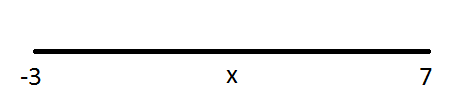is the midpoint of the number line that extends fromto. What is the value of?Explanation:

Find the average of the two endpoints to find their midpoint. To find the average of two numbers, add them together and divide by two.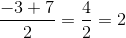### Example Question #2 : How To Find Symmetry Of A Line

How many lines of symmetry does a square have?Explanation:

A line of symmetry is the imaginary line that you draw through a shape so that you can fold the image over the line and have both halves match exactly. Any regular shape has as many lines of symmetry as it does sides. Since a square has four sides, the correct answer is 4!

### Example Question #3 : How To Find Symmetry Of A Line

How many lines of symmetry does an octagon have?Explanation:

A line of symmetry divides a shape into two equal and identical halves. Any regular shape has as many lines of symmetry as it does sides. Since octagons have eight sides, the correct answer is 8.

### Example Question #4 : How To Find Symmetry Of A Line

How many lines of symmetry does a rectangle have?Explanation:

A line of symmetry is the imaginary line that you draw through a shape so that you can fold the image over the line and have both halves match exactly. Any regular shape has as many lines of symmetry as it does total sides. Since a rectangle has four sides, the correct answer is 4!

### Example Question #5 : How To Find Symmetry Of A Line

The length of a certain line isinches. If this line is cut in half, how long is each half?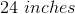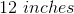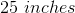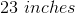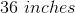Explanation:

Divide the length of the line by 2 to find the length of each half: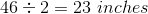### Example Question #6 : How To Find Symmetry Of A Line

How many lines of symmetry does an octagon have?Explanation:

A line of symmetry is the imaginary line that you draw through a shape so that you can fold the image over the line and have both halves match exactly. Any regular shape has as many lines of symmetry as it does sides. Since an octagon has eight sides, the correct answer is.

### Example Question #1 : How To Find Symmetry

Ms. Johnson is knitting a blanket for her grandson. She has a piece of yarn that is exactly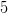feet long however, she only needs half of the piece of yarn. What is the length of the piece of yarn that Ms. Johnson needs to make the blanket for her grandson?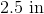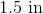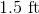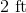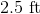Explanation:

Ms. Johnson started withfeet of yarn, however she only needs half of thefeet.

To find the length of the amount of yarn that she needs, dividein half.

The solution is: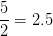### Example Question #2 : How To Find Symmetry

Mr. Thomas' rectangular garden has a width of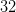feet. The length of his garden is exactly half the distance of the width of the garden. How long is the length of Mr. Thomas' garden?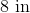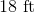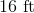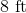Explanation:

The length of Mr. Thomas' garden is exactly half the distance of the width of the garden.

The width of the garden isfeet.

Thus, the length is equal to,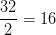### Example Question #3 : How To Find Symmetry

Celeste is Debra's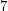year old daughter. Both Debra and Celeste measured their height on the wall. Celeste was surprised to find out that she is exactly half the height of her mother. If Debra is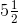feet tall, how tall is Celeste?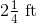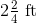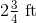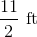Explanation:

Debra is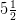feet tall. Celeste is half the height of her mother Debra.

Thus, to find Celeste's height, dividein half.

The solution is:

Step one: convertinto an improper fraction.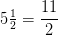Step two: divide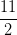by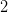.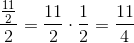Step three: express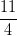in feet as a mixed number.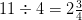### Example Question #4 : How To Find Symmetry

Mr. Lam is planning on painting a rectangular wall in his living room. Before he starts painting, he decided to take a few measurements of the wall. He finds out that the width of the wall is twice the measurement of the height of the wall. If the width of the wall is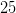feet, what is the height of the wall?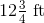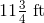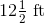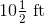Explanation:

Since the height of the rectangular wall is half the length of the width, divideby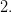The solution is: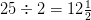Check: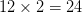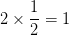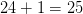← Previous 1 3 4 5 6